plastimatch
Hausdorff_distance Class Reference

The Hausdorff class computes the worst-case distance between two regions. There are many variants of the Hausdorff. See for example: More...

#include <hausdorff_distance.h>

## Public Member Functions

Hausdorff_distance ()

~Hausdorff_distance ()

Inputs
void set_reference_image (const char *image_fn)
Set the reference image. The image will be loaded from the specified filename. More...

void set_reference_image (const UCharImageType::Pointer image)
Set the reference image as an ITK image. More...

void set_compare_image (const char *image_fn)
Set the compare image. The image will be loaded from the specified filename. More...

void set_compare_image (const UCharImageType::Pointer image)
Set the compare image as an ITK image. More...

void set_hausdorff_distance_fraction (float hausdorff_distance_fraction)
Set the fraction of voxels to include when computing the percent hausdorff distance. The input value should be a number between 0 and 1. The default value is 0.95. More...

void set_distance_map_algorithm (const std::string &dmap_alg)
Choose which distance map algorithm to use. More...

void set_maximum_distance (float maximum_distance)
Choose the maximum distance that is returned when computing the distance map. More...

void set_volume_boundary_behavior (Volume_boundary_behavior vbb)

Execution
void run ()
Compute hausdorff distances. More...

Outputs
float get_hausdorff ()
Return the Hausdorff distance. More...

float get_min_min_hausdorff ()
Return the minimum Hausdorff distance. More...

float get_avg_average_hausdorff ()
Return the average Hausdorff distance. More...

float get_max_average_hausdorff ()
Return the max average Hausdorff distance. More...

float get_percent_hausdorff ()
Return the percent Hausdorff distance. More...

float get_boundary_hausdorff ()
Return the boundary Hausdorff distance. More...

float get_min_min_boundary_hausdorff ()
Return the minimum boundary Hausdorff distance. More...

float get_avg_average_boundary_hausdorff ()
Return the average boundary Hausdorff distance. More...

float get_max_average_boundary_hausdorff ()
Return the max average boundary Hausdorff distance. More...

float get_percent_boundary_hausdorff ()
Return the percent boundary Hausdorff distance. More...

void debug ()
Display debugging information to stdout. More...

## Public Attributes

Hausdorff_distance_private * d_ptr

## Protected Member Functions

void run_internal (UCharImageType::Pointer image1, UCharImageType::Pointer image2)

## Detailed Description

The Hausdorff class computes the worst-case distance between two regions. There are many variants of the Hausdorff. See for example:

"A Modified Hausdorff Distance for Object Matching," MP Dubuisson and AK Jain, Proc. International Conference on Pattern Recognition, pp 566–568, 1994.

To understand the variants, we first define the directed Hausdorff and the boundary Hausdorff.

The directed Hausdorff measure from X to Y is defined as the maximum distance, for all points in X, to the closest point in Y. Mathematically this is given as: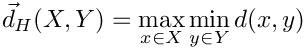The (undirected) Hausdorff distance is the maximum of the two directed Hausdorff measures.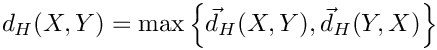The (directed or undirected) boundary Hausdorff is a Hausdorff distance which is computed on the boundary of a set rather than the set itself. For example, the (undirected) boundary Hausdorff distance is given as: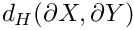The directed average Hausdorff measure is the average distance of a point in X to its closest point in Y. That is:The (undirected) average Hausdorff measure is the average of the two directed average Hausdorff measures: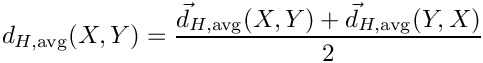The (undirected) max average Hausdorff measure is the maximum of the two directed average Hausdorff measures: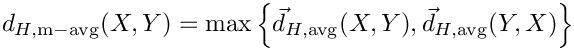The directed percent Hausdorff measure, for a percentile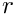, is theth percentile distance over all distances from points in X to their closest point in Y. For example, the directed 95% Hausdorff distance is the point in X with distance to its closest point in Y is greater or equal to exactly 95% of the other points in X. In mathematical terms, denoting theth percentile as, this is given as: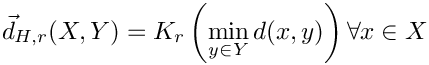The (undirected) percent Hausdorff measure is defined again with the mean: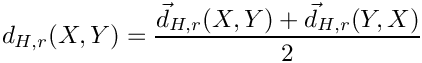If the images do not have the same size and resolution, the compare image will be resampled onto the reference image geometry prior to comparison.

## ◆ Hausdorff_distance()

 Hausdorff_distance::Hausdorff_distance ( )

## ◆ ~Hausdorff_distance()

 Hausdorff_distance::~Hausdorff_distance ( )

## ◆ debug()

 void Hausdorff_distance::debug ( void )

Display debugging information to stdout.

## ◆ get_avg_average_boundary_hausdorff()

 float Hausdorff_distance::get_avg_average_boundary_hausdorff ( )

Return the average boundary Hausdorff distance.

## ◆ get_avg_average_hausdorff()

 float Hausdorff_distance::get_avg_average_hausdorff ( )

Return the average Hausdorff distance.

## ◆ get_boundary_hausdorff()

 float Hausdorff_distance::get_boundary_hausdorff ( )

Return the boundary Hausdorff distance.

## ◆ get_hausdorff()

 float Hausdorff_distance::get_hausdorff ( )

Return the Hausdorff distance.

## ◆ get_max_average_boundary_hausdorff()

 float Hausdorff_distance::get_max_average_boundary_hausdorff ( )

Return the max average boundary Hausdorff distance.

## ◆ get_max_average_hausdorff()

 float Hausdorff_distance::get_max_average_hausdorff ( )

Return the max average Hausdorff distance.

## ◆ get_min_min_boundary_hausdorff()

 float Hausdorff_distance::get_min_min_boundary_hausdorff ( )

Return the minimum boundary Hausdorff distance.

## ◆ get_min_min_hausdorff()

 float Hausdorff_distance::get_min_min_hausdorff ( )

Return the minimum Hausdorff distance.

## ◆ get_percent_boundary_hausdorff()

 float Hausdorff_distance::get_percent_boundary_hausdorff ( )

Return the percent boundary Hausdorff distance.

## ◆ get_percent_hausdorff()

 float Hausdorff_distance::get_percent_hausdorff ( )

Return the percent Hausdorff distance.

## ◆ run()

 void Hausdorff_distance::run ( )

Compute hausdorff distances.

## ◆ run_internal()

 void Hausdorff_distance::run_internal ( UCharImageType::Pointer image1, UCharImageType::Pointer image2 )
protected

## ◆ set_compare_image() [1/2]

 void Hausdorff_distance::set_compare_image ( const char * image_fn )

Set the compare image. The image will be loaded from the specified filename.

## ◆ set_compare_image() [2/2]

 void Hausdorff_distance::set_compare_image ( const UCharImageType::Pointer image )

Set the compare image as an ITK image.

## ◆ set_distance_map_algorithm()

 void Hausdorff_distance::set_distance_map_algorithm ( const std::string & dmap_alg )

Choose which distance map algorithm to use.

## ◆ set_hausdorff_distance_fraction()

 void Hausdorff_distance::set_hausdorff_distance_fraction ( float hausdorff_distance_fraction )

Set the fraction of voxels to include when computing the percent hausdorff distance. The input value should be a number between 0 and 1. The default value is 0.95.

## ◆ set_maximum_distance()

 void Hausdorff_distance::set_maximum_distance ( float maximum_distance )

Choose the maximum distance that is returned when computing the distance map.

## ◆ set_reference_image() [1/2]

 void Hausdorff_distance::set_reference_image ( const char * image_fn )

Set the reference image. The image will be loaded from the specified filename.

## ◆ set_reference_image() [2/2]

 void Hausdorff_distance::set_reference_image ( const UCharImageType::Pointer image )

Set the reference image as an ITK image.

## ◆ set_volume_boundary_behavior()

 void Hausdorff_distance::set_volume_boundary_behavior ( Volume_boundary_behavior vbb )